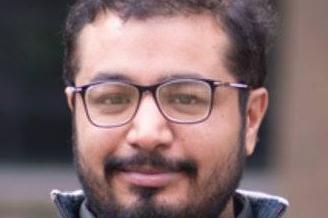Post Date
Nov 29 2023

## Functional Partial Differential Equations

Year
2022
Supervisor:
Dr. Ali Ashher Zaidi
Students:
Syed Touqeer Hussain Shah
MS/PhD
PhD
Reference / Filters
Mathematics

Abstract:

The thesis concerns the development of the theory of functional (nonlocal) partial differential equations (PDEs). Such equations arise in various applications including size structured cell growth models, collection of current in an electric train, light absorption in the Milky Way, fragmentation in polymers, droplets, telecommunication systems to describe certain internet protocols, and more recently granular Physics. The equations are supplemented with appropriate initial and boundary conditions. There are no general methods for solving such problems due to the presence of nonlocal terms in the equation.

We first consider an initial boundary value problem that involves a functional partial differential equation with two nonlocal terms and a broad class of coefficients. We establish the existence of an attracting solution, towards which solutions to the problem converge for large time.

We then devise a method of solving an initial boundary value problem that involves a second-order parabolic partial differential equation with two nonlocal terms and constant coefficients. The presence of two nonlocal terms is a consequence of asymmetry in cell division. The solution techniques for solving such problems are rare due to nonlocal terms. We obtain separable solutions, as well as the general solution to the full problem, and show that the solutions to the problem converge to the separable solution for large time. The dispersion term does not affect the rate of convergence to the separable solution.

We then establish the existence of a unique solution to an initial boundary value problem that involves a non-homogeneous functional partial differential equation of the pantograph type with singular time dependent coefficients. The problem is also motivated from a cell division equation.

We also study an initial boundary value problem that involves a functional partial differential equation with two nonlocal terms and a certain class of time dependent coefficients. We establish the existence of a large time attracting solution at least for a certain class of coefficients. The rate of convergence of solutions towards the large time attracting solution is affected by the choice of coefficients and remains unaffected by the number of nonlocal terms. The uniqueness of solutions to the initial boundary value problem is also established.

Publications:

1. STH Shah, AA Zaidi, The existence of solutions to an inhomogeneous pantograph type equation with singular coefficients, Journal of Elliptic and Parabolic Equations, (2020) 6:935-945 DOI 10.1007/s41808-020-00089-3

2. M Efendiev, B van Brunt, AA Zaidi, STH Shah, Asymmetric cell division with stochastic growth rate. Dedicated to the memory of the late Spartak Agamirzayev, Mathematical Methods in the Applied Sciences (2018), 41 (17), 8059-8069

3. AA Zaidi, STH Shah, A cell division equation with time dependent growth and division rates, Under review, 2021.